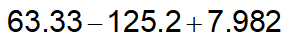# Problem: Perform the following calculation to the right number of sig figs:

###### Problem Details

Perform the following calculation to the right number of sig figs:Frequently Asked Questions

What scientific concept do you need to know in order to solve this problem?

Our tutors have indicated that to solve this problem you will need to apply the Addition and Subtraction Operations concept. You can view video lessons to learn Addition and Subtraction Operations. Or if you need more Addition and Subtraction Operations practice, you can also practice Addition and Subtraction Operations practice problems.

What is the difficulty of this problem?

Our tutors rated the difficulty ofPerform the following calculation to the right number of sig...as low difficulty.

How long does this problem take to solve?

Our expert Analytical Chemistry tutor, Jules took undefined 47 seconds to solve this problem. You can follow their steps in the video explanation above.